# 1 Wednesday, January 13

Reference:

## 1.1 Overview

A complex is given by \begin{align*} \cdots \xrightarrow{d_{i-1}} M_{i-1} \xrightarrow{d_i} M_i \xrightarrow{d_{i+1}}M_{i+1} \to \cdots .\end{align*} where $$M_i \in {\mathsf{R}{\hbox{-}}\mathsf{Mod}}$$ and $$d_i \circ d_{i-1} = 0$$, which happens if and only if $$\operatorname{im}d_{i-1} \subseteq \ker d_i$$. If $$\operatorname{im}d_{i-1} = \ker d_i$$, this complex is exact.

We can apply a functor such as $$\otimes_R N$$ to get a new complex \begin{align*} \cdots \xrightarrow{d_{i-1} \otimes 1_N} M_{i-1} \otimes_R N \xrightarrow{d_i \otimes 1} M_i \otimes N \to M_{i+1} \xrightarrow{d_{i+1} \otimes 1} \cdots .\end{align*}

Applying $$\mathop{\mathrm{Hom}}(N, {-})$$ similarly yields \begin{align*} \mathop{\mathrm{Hom}}_R(N, M_{i}) \xrightarrow{d_{i-1}^*} \mathop{\mathrm{Hom}}_R(N, M_{i+1}) ,\end{align*} where $$d_i^* = d_i \circ ({-})$$ is given by composition.

Applying $$\mathop{\mathrm{Hom}}({-}, N)$$ yields \begin{align*} \mathop{\mathrm{Hom}}_R(M_i, N) \xrightarrow{d_{i}^*} \mathop{\mathrm{Hom}}_R(M_{i+1}, N) \end{align*} where $$d_i^* = ({-}) \circ d_i$$.

Note that we can also take complexes with arrows in the other direction. For $$F$$ a functor, we can rewrite these examples as \begin{align*} d_i^* \circ d_{i-1}^* = F(d_i) \circ F(d_{i-1}) = F(d_i \circ d_{i-1}) = F(0) = 0 ,\end{align*} provided $$F$$ is nice enough and sends zero to zero. This follows from the fact that functors preserve composition. Even if the original complex is exact, the new one may not be, so we can define the following:

\begin{align*} H^i(M^*) = \ker d_i^* / \operatorname{im}d_{i-1}^* .\end{align*}

These will lead to $$i$$th derived functors, and category theory will be useful here. See appendix in Weibel. For a category $$\mathcal{C}$$ we’ll define

• $$\mathrm{Obj}(\mathcal{C} )$$ as the objects
• $$\mathop{\mathrm{Hom}}_{\mathcal{C}}(A, B)$$ a set of morphisms between them, where a more modern notation might be $$\mathrm{Mor}(A, B)$$.
• Morphisms compose: $$A \xrightarrow{f} B \xrightarrow{g} C$$ means that $$g\circ f \in \mathop{\mathrm{Hom}}_{\mathcal{C}}(A, C)$$
• Associativity
• Identity morphisms

See the appendix for diagrams defining zero objects and the zero map, which we’ll need to make sense of exactness. We’ll also needs notions of kernels and images, or potentially cokernels instead of images since they’re closely related.

In the examples, we had $$\ker d_i \subseteq M_i$$, but this need not be true since the objects in the category may not be sets. Such an example is the category of complexes of $$R{\hbox{-}}$$modules: $$\operatorname{Cx}({\mathsf{R}{\hbox{-}}\mathsf{Mod}})$$. In this setting, kernels will be subcomplexes but not subsets.

Recall that functors are “functions” between categories $$F: \mathcal{C}\to \mathcal{D}$$ such that

• Objects are sent to objects,

• Morphisms are sent to morphisms, so $$A \xrightarrow{f} B \leadsto F(A) \xrightarrow{F(f)} F(B)$$,

• $$F$$ respects composition and identities

$$\mathop{\mathrm{Hom}}_R(N, {-}): {\mathsf{R}{\hbox{-}}\mathsf{Mod}}\to {\mathsf{Ab}}$$, noting that the hom set may not have an $$R{\hbox{-}}$$module structure.

Taking cohomology yields the $$i$$th derived functors of $$F$$, for example $$\operatorname{Ext}^i, \operatorname{Tor}_i$$. Recall that functors can be covariant or contravariant. See section 1 for formulating simplicial and singular homology (from topology) in this language.

## 1.2 Chapter 1: Chain Complexes

### 1.2.1 Complexes of $$R{\hbox{-}}$$modules

Let $$R$$ be a ring with 1 and define $${\mathsf{R}{\hbox{-}}\mathsf{Mod}}$$ to be the category of right $$R{\hbox{-}}$$modules. $$A \xrightarrow{f} B \xrightarrow{g} C$$ is exact if and only if $$\ker g = \operatorname{im}f$$, and in particular $$g\circ f = 0$$.

A chain complex is \begin{align*} C_{-}\mathrel{\vcenter{:}}=(C_{-}, d_{-}) \mathrel{\vcenter{:}}=\qty{ \cdots \to C_{n+1} \xrightarrow{d_{n+1}} C_n \xrightarrow{d_n} C_{n-1} \to \cdots } \end{align*} for $$n \in {\mathbb{Z}}$$ such that $$d_n \circ d_{n+1} = 0$$. We drop the $$n$$ from the notation and write $$d^2 \mathrel{\vcenter{:}}= d\circ d = 0$$.

• $$Z_n = Z_n(C_{-}) = \ker d_n$$ are referred to as $$n{\hbox{-}}$$cycles.
• $$B_n = B_n(C_{-}) = \operatorname{im}d_{n+1}$$ are the $$n{\hbox{-}}$$boundaries.

Note that if $$d^2 = 0$$ then $$B_n \leq Z_n \leq C_n$$. In this case, it makes sense to define the quotient module $$H^n(C_{-}) \mathrel{\vcenter{:}}= Z_n / B_n$$, the $$n$$th homology of $$C_{-}$$.

A map $$u: C_{-}\to D_{-}$$ of chain complexes is a sequence of maps $$u_n: C_n \to D_n$$ such that all of the following squares commute:Link to Diagram

We can thus define a category $$\mathrm{Ch}({\mathsf{R}{\hbox{-}}\mathsf{Mod}})$$ where

• The objects are chain complexes,
• The morphisms are chain maps.

A chain complex map $$u: C_{-}\to D_{-}$$ restricts to \begin{align*} u_n: Z_n(C_{-}) \to Z_n(D_{-}) \\ u_n: B_n(D_{-}) \to B_n(D_{-}) \end{align*} and thus induces a well-defined map $$u_{n, *}: H_n(C_{-}) \to H_n(D_{-})$$.

Each $$H_n$$ thus becomes a functor $$\mathrm{Ch}({\mathsf{R}{\hbox{-}}\mathsf{Mod}}) \to {\mathsf{R}{\hbox{-}}\mathsf{Mod}}$$ where $$H_n(u) \mathrel{\vcenter{:}}= u_{*. n}$$.

# 2 Friday, January 15

## 2.1 Review

See assignment posted on ELC, due Wed Jan 27

Recall that a chain complex is $$C_{-}$$ where $$d^2 = 0$$, and a map of chain complex is a ladder of commuting squaresLink to diagram

Recall that $$u_n: Z_n(C) \to Z_n(D)$$ and $$u_n: B_n(C) \to B_n(D)$$ preserves these submodules, so there are induced maps $$u_{{-}, n}: H_n(D) \to H_n(D)$$ where $$H_n(C) \mathrel{\vcenter{:}}= Z_n(C) / B_nn-1(C)$$. Moreover, taking $$H_n({-})$$ is a functor from $$\mathsf{Ch}({\mathsf{R}{\hbox{-}}\mathsf{Mod}}) \to {\mathsf{R}{\hbox{-}}\mathsf{Mod}}$$ for any fixed $$n$$ and on objects $$C\mapsto H_n(C)$$ and chain maps $$u_{n} \to H_n(u) \mathrel{\vcenter{:}}= u_{*, n}$$. Note the lower indices denote maps going down in degree.

## 2.2 Cohomology

A chain map $$u:C\to D$$ is a quasi-isomorphism if and only if the induced map $$u_{*, n}: H^n(C) \to H^n(D)$$ is an isomorphism of $$R{\hbox{-}}$$modules.

Note that the usual notion of an isomorphism in the categorical sense might be too strong here.

A cochain complex is a complex of the form \begin{align*} \cdots \xrightarrow{d^{n-2}} C^{n-1} \xrightarrow{d^{n-1}} C^{n} \xrightarrow{d^{n}} C^{n+1} \cdots \end{align*} where $$d^n \circ d^{n-1} = 0$$. We similarly write $$Z^n(C) \mathrel{\vcenter{:}}=\ker d^n$$ and $$B^n(C) \mathrel{\vcenter{:}}=\operatorname{im}d^{n-1}$$ and write the $$R{\hbox{-}}$$module $$H^n(C) \mathrel{\vcenter{:}}= Z^n/B^n$$ for the $$n$$th cohomology of $$C$$.

There is a way to go back and forth bw chain complexes and cochain complexes: set $$C_n \mathrel{\vcenter{:}}= C^{-n}$$ and $$d_n \mathrel{\vcenter{:}}= d^{-n}$$. This yields \begin{align*} C^{-n} \xrightarrow{d^{-n}} C^{-n+1} \iff C_n \xrightarrow{d^n} C_{n-1} ,\end{align*} and the notions of $$d^2 = 0$$ coincide.

A cochain complex $$C$$ is bounded if and only if there exists an $$a\leq b \in {\mathbb{Z}}$$ such that $$C_n \neq 0 \iff a\leq n \leq b$$. Similarly $$C^n$$ is bounded above if there is just a $$b$$, and bounded below for just an $$a$$. All of the same definitions are made for cochain complexes.

See the book for classical applications:

• 1.1.3: Simplicial homology
• 1.1.5: Singular homology

## 2.3 Operations on Chain Complexes

Write $$\mathsf{Ch}$$ for $$\mathsf{Ch}({\mathsf{R}{\hbox{-}}\mathsf{Mod}})$$, then if $$f,g: C\to D$$ are chain maps then $$f+g:C\to D$$ can be defined as $$(f+g)(x) = f(x) + g(x)$$, since $$D$$ has an addition coming from its $$R{\hbox{-}}$$module structure. Thus the hom sets $$\mathop{\mathrm{Hom}}_{\mathsf{Ch}}(C, D)$$ becomes an abelian group. There is a distinguished zero object1 $$0$$, defined as the chain complex with all zero objects and all zero maps. Note that we also have a zero map given by the composition $$(C \to 0) \circ (0\to D)$$.

If $$\left\{{A_ \alpha}\right\}$$ is a family of complexes, we can form two new complexes:

• The product $$\qty{ \prod_ \alpha A_ \alpha}_n \mathrel{\vcenter{:}}=\prod_ \alpha A _{\alpha, n}$$ with the differential \begin{align*} \qty{ \prod d_ \alpha}_n: \prod A _{\alpha, n} \xrightarrow{d _{\alpha, n}} \prod A _{\alpha, n-1} .\end{align*}

• The coproduct $$\qty{ \coprod _{\alpha} A _{\alpha}}_n \mathrel{\vcenter{:}}=\bigoplus _{\alpha} A _{\alpha, n}$$, i.e. there are only finitely many nonzero entries, with exactly the same definition as above for the differential.

Note that if the index set is finite, these notions coincide. By convention, finite direct products are written as direct sums.

These structures make $$\mathsf{Ch}$$ into an additive category. See appendix for definition: the homs are abelian groups where composition distributes over addition, existence of a zero object, and existence of finite products. Note that here we have arbitrary products.

We say $$B$$ is a subcomplex of $$C$$ if and only if

• $$B_n \leq C_n \in {\mathsf{R}{\hbox{-}}\mathsf{Mod}}$$ for all $$n$$,
• The differentials of $$B_n$$ are the restrictions of the differentials of $$C_n$$.

This can be alternatively stated as saying the inclusion $$i: B\to C$$ given by $$i_n: B_n \to C_n$$ is a morphism of chain complexes. Recall that some squares need to commute, and this forces the condition on restrictions.

When $$B \leq C$$, we can form the quotient complex $$C/B$$ where \begin{align*} C_n/B_n \xrightarrow{\mkern 1.5mu\overline{\mkern-1.5mud_n\mkern-1.5mu}\mkern 1.5mu} C _{n-1} / B _{n-1} .\end{align*} Moreover there is a natural projection $$\pi: C\to C/B$$ which is a chain map.

Suppose $$f:B\to C$$ is a chain map, then there exist induced maps on the levelwise kernels and cokernels, so we can form the kernel and cokernel complex: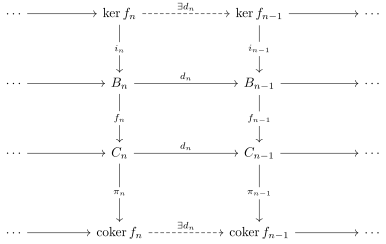Link to Diagram

Here $$\ker f \leq B$$ is a subcomplex, and $$\operatorname{coker}f$$ is a quotient complex of $$C$$. The chain map $$i: \ker f\to B$$ is a categorical kernel of $$f$$ in $$\mathsf{Ch}$$, and $$\pi$$ is similarly a cokernel. See appendix A 1.6. These constructions make $$\mathsf{Ch}$$ into an abelian category: roughly an additive category where every morphism has a kernel and a cokernel.

# 3 1.2: Chain Complex of Chain Complexes (Wednesday, January 20)

See phone pic for missed first 10m

## 3.1 Double Complexes

Consider a double complex: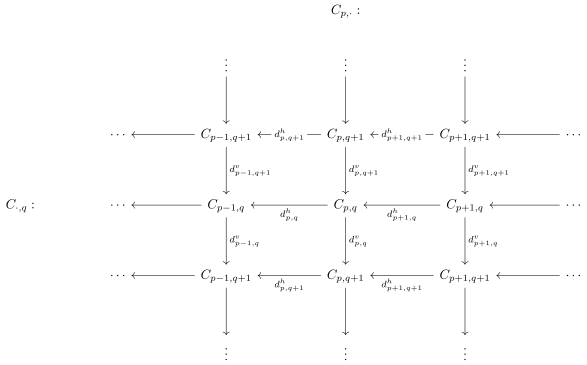Link to Diagram

All of the individual rows and columns are chain complexes, where $$(d^h)^2 = 0$$ and $$(d^v)^2 = 0$$, and the square anticommute: $$d^v d^h + d^h d^v - 0$$, so $$d^v d^h = -d^h d^v$$. This is almost a chain complex of chain complexes, i.e. an element of $$\mathsf{Ch}(\mathsf{Ch}{\mathsf{R}{\hbox{-}}\mathsf{Mod}}))$$. It’s useful here to consider lines parallel to the line $$y=x$$.

A double complex $$C_{{-}, {-}}$$ is bounded if and only if there are only finitely many nonzero terms along each constant diagonal $$p+q = n$$.

A first quadrant double complex $$\left\{{C_{p, q}}\right\}_{p, q\geq 0}$$ is bounded: note that this can still have infinitely many terms, but each diagonal is finite because each will hit a coordinate axis.

The squares anticommute, since the $$d^v$$ are not chain maps between the horizontal chain complexes. This can be fixed by changing every one out of four signs, defining \begin{align*} f_{*, q}: C_{*, q} \to C_{*, q-1} \\ f_{p, q} \mathrel{\vcenter{:}}=(-1)^p d^v_{p, q}: C_{p,q} \to C_{p, q-1} .\end{align*}

This yields a new double complex where the signs of each column alternate: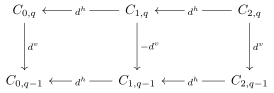Now the squares commute and $$f_{{-}, q}$$ are chain maps, so this object is an element of $$\mathsf{Ch}(\mathsf{Ch}{\mathsf{R}{\hbox{-}}\mathsf{Mod}})$$.

## 3.2 Total Complexes

Recall that products and coproducts of $$R{\hbox{-}}$$modules coincide when the indexing set is finite.

Given a double complex $$C_{{-}, {-}}$$, there are two ordinary chain complexes associated to it referred to as total complexes: \begin{align*} (\operatorname{Tot}^{\Pi}C)_n &\mathrel{\vcenter{:}}=\prod_{p+q = n} C_{p, q}\\ (\operatorname{Tot}^{\oplus}C)_n &\mathrel{\vcenter{:}}=\bigoplus_{p+q = n} C_{p, q} .\end{align*} Writing $$\operatorname{Tot}(C)$$ usually refers to the former. The differentials are given by \begin{align*} d_{p, q} = d^h + d^v: C_{p, q} \to C_{p-1, q} \oplus C_{p, q-1} ,\end{align*} where $$C_{p, q} \subseteq \operatorname{Tot}^{\oplus}(C)_n$$ and $$C_{p-1, q} \oplus C_{p, q-1} \subseteq \operatorname{Tot}^{\oplus}(C)_{n-1}$$. Then you extend this to a differential on the entire diagonal by defining $$d = \bigoplus_{p, q} d_{p, q}$$.

Check that $$d^2 = 0$$, using $$d^v d^h + d^h d^v = 0$$.

Some notes:

• $$\operatorname{Tot}^{\oplus}(C) = \operatorname{Tot}^{\Pi}(C)$$ when $$C$$ is bounded.

• The total complexes need not exist if $$C$$ is unbounded: one needs infinite direct products and infinite coproducts to exist in $$\mathcal{C}$$. A category admitting these is called complete or cocomplete.2

## 3.3 More Operations

Fix $$n\in {\mathbb{Z}}$$, and define the $$n$$th truncation $$\tau_{\geq n}(C)$$ by \begin{align*} \tau_{\geq n}(C) = \begin{cases} 0 & i < n \\ Z_n & i= n \\ C_i & i > n . \end{cases} .\end{align*}

Pictorially: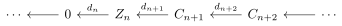Link to diagram

This is sometimes call the good truncation of $$C$$ below $$n$$.

Note that \begin{align*} H_i(\tau_{\geq n} C) = \begin{cases} 0 & i < n \\ H_i(C) & i\geq n. \end{cases} .\end{align*}

We define the quotient complex \begin{align*} \tau_{ which is $$C_i$$ below $$n$$, $$C_n/Z_n$$ at $$n$$. Thus is has homology \begin{align*} \begin{cases} H_i(C) & i< n. \\ 0 & i \geq n \end{cases} .\end{align*}

If $$C$$ is a chain complex and $$p\in {\mathbb{Z}}$$, define a new complex $$C[p]$$ by \begin{align*} C[p]_n \mathrel{\vcenter{:}}= C_{n+p} .\end{align*}Link to Diagram

Similarly, if $$C$$ is a cochain complex, we set $$C[p]^n \mathrel{\vcenter{:}}= C^{n-p}$$:Link to Diagram

Mnemonic: Shift $$p$$ positions in the same direction as the arrows.

In both cases, the differentials are given by the shifted differential $$d[p] \mathrel{\vcenter{:}}=(-1)^p d$$. Note that these are not alternating: $$p$$ is the fixed translation, so this is a constant that changes the signs of all differentials. Thus $$H_n(C[p]) = H_{n+p}(C)$$ and $$H^n(C[p]) = H^{n-p}$$.

Check that if $$C^n \mathrel{\vcenter{:}}= C_{-n}$$, then $$C[p]^n = C[p]_{-n}$$.

We can make translation into a functor $$[p]: \mathsf{Ch}\to \mathsf{Ch}$$: given $$f: C\to D$$, define $$f[p]: C[p] \to D[p]$$ by $$f[p]_n \mathrel{\vcenter{:}}= f_{n+p}$$, and a similar definition for cochain complexes changing $$p$$ to $$-p$$.

# 4 Lecture 4 (Friday, January 22)

## 4.1 Long Exact Sequences

Some terminology: in an abelian category $$\mathcal{A}$$ an example of an exact complex in $$\mathsf{Ch}(\mathcal{A})$$ is \begin{align*} \cdots \to 0 \to A \xrightarrow{f} B \xrightarrow{g} C \to 0 \to \cdots .\end{align*}

where exactness means $$\ker = \operatorname{im}$$ at each position, i.e. $$\ker f = 0, \operatorname{im}f = \ker g, \operatorname{im}g = C$$. We say $$f$$ is monic and $$g$$ epic.

As a special case, if $$0\to A\to 0$$ is exact then $$A$$ must be zero, since the image of the incoming map must be 0. This also happens when every other term is zero. If $$0\to A \xrightarrow{f} B \to 0$$, then $$A \cong B$$ since $$f$$ is both injective and surjective (say for $$R{\hbox{-}}$$modules).

Suppose $$0\to A\to B \to C \to 0$$ is a SES in $$\mathsf{Ch}(\mathcal{A})$$ (note: this is a sequence of complexes), then there are natural maps \begin{align*} \delta: H_n(C) \to H_{n-1}(A) \end{align*} called connecting morphisms which decrease degree such that the following sequence is exact:Link to Diagram

This is referred to as the long exact sequence in homology. Similarly, replacing chain complexes by cochain complexes yields a similar connecting morphism that increases degree.

Note on notation: some books use $${{\partial}}$$ for homology and $$\delta$$ for cohomology.

The proof that this sequence exists is a consequence of the snake lemma.

The sequence highlighted in red in the following diagram is exact: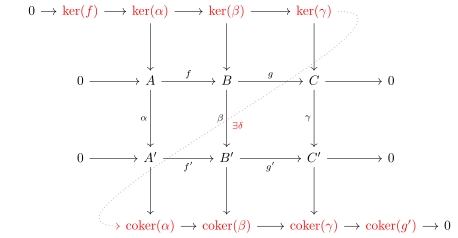Link to Diagram

• Start with $$c\in \ker(\gamma) \leq C$$, so $$\gamma(c) = 0 \in C'$$
• Choose $$b\in B$$ by surjectivity
• We’ll show it’s independent of this choice.
• Then $$b'\in B'$$ goes to $$0\in C'$$, so $$b' \in \ker (B' \to C')$$
• By exactness, $$b' \in \ker (B' \to C') = \operatorname{im}(A'\to B')$$, and now produce a unique $$a'\in A'$$ by injectivity
• Take the image $$[a']\in \operatorname{coker}\alpha$$
• Define $${{\partial}}(c) \mathrel{\vcenter{:}}=[a']$$.
• We chose $$b$$, suppose we chose a different $$\tilde b$$.
• Then $$\tilde b - b \mapsto c-c = 0$$, so the difference is in $$\ker g = \operatorname{im}f$$.
• Produce an $$\tilde a\in A$$ such that $$\tilde a\mapsto \tilde b - b$$
• Then $$\mkern 1.5mu\overline{\mkern-1.5mua\mkern-1.5mu}\mkern 1.5mu \mathrel{\vcenter{:}}=\alpha(\tilde a)$$, so apply $$f'$$.
• Define $$\beta(\tilde b) = \tilde b ' \in B$$.
• Commutativity of the LHS square forces $$\tilde a'\mapsto \tilde b' - b'$$.
• Then $$\mkern 1.5mu\overline{\mkern-1.5mua\mkern-1.5mu}\mkern 1.5mu + a' \mapsto \tilde b' -b' + b' = \tilde b'$$.
• So $$\tilde a' + a'$$ is the desired pullback of $$\tilde b'$$
• Then take $$[\tilde a'] \in \operatorname{coker}\alpha$$; are $$a', \tilde a'$$ in the same equivalence class?
• Use that fact that $$\tilde a = a' + \mkern 1.5mu\overline{\mkern-1.5mua\mkern-1.5mu}\mkern 1.5mu$$, where $$\mkern 1.5mu\overline{\mkern-1.5mua\mkern-1.5mu}\mkern 1.5mu \in \operatorname{im}\alpha$$, so $$[\tilde a] = [a' + \mkern 1.5mu\overline{\mkern-1.5mua\mkern-1.5mu}\mkern 1.5mu] = [a'] \in \operatorname{coker}\alpha \mathrel{\vcenter{:}}= A'/\operatorname{im}\alpha$$.
• Let’s show $$g: \ker \beta\to \ker \gamma$$.

• Let $$b \in \ker \beta$$, then consider $$\gamma(g(\beta)) = g'(\beta(b)) = g'(0) = 0$$ and so $$g(b) \in \ker \gamma$$.
• Now we’ll show $$\operatorname{im}({ \left.{{g}} \right|_{{\ker \beta}} }) \subseteq \ker \delta$$

• Let $$b \in \ker \beta, c = g(b)$$, then how is $$\delta(c)$$ defined?
• Use this $$b$$, then apply $$\beta$$ to get $$b' = \beta(b) = 0$$ since $$b \in \ker \beta$$.
• So the unique thing mapping to it $$a'$$ is zero, and thus $$[a'] = 0 = \delta(c)$$.
• $$\ker \delta \subseteq \operatorname{im}( { \left.{{g}} \right|_{{ \ker \beta}} } )$$

• Let $$c\in \ker \delta$$, then $$\delta(c) = 0 = [a'] \in \operatorname{coker}\alpha$$ which implies that $$a' \in \operatorname{im}\alpha$$.
• Write $$a' = \alpha(a)$$, then $$\beta(b) = b' = f'(a') = f'( \alpha(a))$$ by going one way around the LHS square, and is equal to $$\beta(f(a))$$ going the other way.
• So $$\tilde b \mathrel{\vcenter{:}}= b - f(a) \in \ker \beta$$, since $$\beta(b) = \beta(f(a))$$ implies their difference is zero.
• Then $$g(\tilde b) = g(b) - g(f(a)) = g(b) = c$$, which puts $$c\in g(\ker \beta)$$ as desired.

Show exactness at the remaining places – the most interesting place is at $$\operatorname{coker}\alpha$$. Also check that all of these maps make sense.

We assumed that $$\mathcal{A}= {\mathsf{R}{\hbox{-}}\mathsf{Mod}}$$ here, so we could chase elements, but this happens to also be true in any abelian category $$\mathcal{A}$$ but by a different proof. The idea is to embed $$\mathcal{A} \to {\mathsf{R}{\hbox{-}}\mathsf{Mod}}$$ for some ring $$R$$, do the construction there, and pull the results back – but this doesn’t quite work! $$\mathcal{A}$$ can be too big. Instead, do this for the smallest subcategory $$\mathcal{A}_0$$ containing all of the modules and maps involved in the snake lemma. Then $$\mathcal{A}_0$$ is small enough to embed into $${\mathsf{R}{\hbox{-}}\mathsf{Mod}}$$ by the Freyd-Mitchell Embedding Theorem.

# 5 Lecture 5 (Monday, January 25)

## 5.1 LES Associated to a SES

For every SES of chain complexes, there is a long exact sequence in homology.

Suppose we have a SES of chain complexes \begin{align*} 0 \to A \xrightarrow{f} B \xrightarrow{g} C \to 0 ,\end{align*} which means that for every $$n$$ there is a SES of $$R{\hbox{-}}$$modules. Recall the diagram for the snake lemma, involving kernels across the top and cokernels across the bottom. Applying the snake lemma, by hypothesis $$\operatorname{coker}g = 0$$ and $$\ker f = 0$$. There is a SES

\begin{align*} A_n / d A_{n+1} \to B_n / d B_{n+1} \to C_n / d C_{n+1} \to 0 \end{align*}

Using the fact that $$B_n \subseteq Z_n$$, we can use the 1st and 2nd isomorphism theorems to produceLink to diagram

This yields an exact sequence relating $$H_n$$ to $$H_{n-1}$$, and these can all be spliced together.

• $$\ker(A_n / d A_{n-1} \to Z_{n-1}(A) = Z_n(A) / d A_{n+1} \mathrel{\vcenter{:}}= H_n(A)$$ using the 2nd isomorphism theorem

Note that $$d$$ is natural, which means the following: there is a category $$\mathcal{S}$$ whose objects are SESs of chain complexes and whose maps are chain maps:There is another full subcategory $$\mathcal{L}$$ of $$\mathsf{Ch}$$ whose objects are LESs of objects in the original abelian category, i.e. exact chain complexes. The claim is that the LES construction in the theorem defines a functor $$\mathcal{S}\to \mathcal{L}$$. We’ve seen how this maps objects, so what is the map on morphisms? Given a morphism as in the above diagram, there is an induced morphism:Link to Diagram

The first two squares commute, and naturality means that the third square commutes as well.

Check the details!

It is sometimes useful to explicitly know how to compute snake lemma boundary elements. See the book for a recipe for computing $${{\partial}}(\xi)$$:

• Lift $$\xi$$ to a cycle $$c\in Z_n(C) \subseteq C_n$$.
• Pull $$c$$ back to a preimage $$b\in B_n$$ by surjectivity.
• Apply the differential to get $$d(b)\in Z_{n-1}(B)$$, using that images are contained in kernels.
• Since this is in kernel of the outgoing map, it’s in the kernel of the incoming map and thus there exists an $$a\in Z_{n-1}(A)$$ such that $$f(a) = db$$
• So set $$\delta(\xi) \mathrel{\vcenter{:}}=[a] \in H_{n-1}(A)$$.

Why is naturality useful? Suppose $$H_n(B) = 0$$, you get isomorphisms, and this allows inductive arguments up the LES. The LES in homology is sometimes abbreviated as an exact triangle:Here $${{\partial}}:H_*(C) \to H_*(A)$$ shifts degrees. Note that this motivates the idea of triangulated categories, which is important in modern research. See Weibel Ch.10, and exercise 1.4.5 for how to construct these as quotients of $$\mathsf{Ch}$$.

## 5.2 1.4: Chain Homotopies

Assume for now that we’re in the situation of $$R{\hbox{-}}$$modules where $$R$$ is a field, i.e. vector spaces. The main fact/advantage here that is not generally true for $$R{\hbox{-}}$$modules: every subspace has a complement. Since $$B_n \subseteq Z_n \subseteq C_n$$, we can write $$C_n = Z_n \oplus B_n'$$ for every $$n$$, and $$Z_n = B_n \oplus H_n$$. This notation is suggestive, since $$H_n \cong Z_n/B_n$$ as a quotient of vector spaces. Substituting, we get $$C_n = B_n \oplus H_n \oplus B_n'$$. Consider the projection $$C_n \to B_n$$ by projecting onto the first factor. Identifying $$B_n \mathrel{\vcenter{:}}=\operatorname{im}(C_{n+1} \to C_n) \cong C_{n+1}/Z_{n+1}$$ by the 1st isomorphism theorem in the reverse direction. But this image is equal to $$B_{n+1}'$$, and we can embed this in $$C_{n+1}$$, so define $$s_n: C_n \to C_{n+1}$$ as the composition \begin{align*} s_n \mathrel{\vcenter{:}}=( C_n \xrightarrow{\mathop{\mathrm{proj}}} B_n = \operatorname{im}(C_{n+1} \to C_n) \xrightarrow{d_{n+1}^{-1}} C_{n+1}/Z_{n+1} \xrightarrow{\cong} B_{n+1}' \hookrightarrow C_{n+1} .\end{align*}

$$d_{n+1} s_n d_{n+1} = d_{n+1}$$ are equal as maps.

• Check on the first factor $$B_{n+1}' \subseteq C_{n+1}$$ directly to get $$s_n d_{n+1}(x) = d_{n+1}(x)$$ for $$x\in B_{n+1}'$$, and then applying $$d_{n+1}$$ to both sides is the desired equality.
• On the second factor $$Z_{n+1}$$, both sides give zero since this is exactly the kernel.

$$d_{n+1} s_n + s_{n-1}d_n = \operatorname{id}_{C_n}$$ if and only if $$H_n = 0$$, i.e. the complex $$C$$ is exact at $$C_n$$. This map is the sum of taking the two triangle paths in this diagram:We again check this on both factors:

• Using the first claim, $$s_n = 0$$ on $$B_n'$$ and thus $$s_{n-1} d_n = \operatorname{id}_{B_n'}$$.

• On $$H_n$$, $$s_n = 0$$ and $$d_n = 0$$, and so the LHS is $$0 = \operatorname{id}_{H_n}$$ if and only if $$H_n = 0$$.

• On $$B_n$$, and tracing through the definition of $$s_n$$ yields $$d_{n+1} s_n(x) = x$$ and this yields $$\operatorname{id}_{B_n}$$.

Next time: summary of decompositions, start general section on chain homotopies.

# 6 Wednesday, January 27

See phone pic for missed first 10m.

## 6.1 1.4: Chain Homotopies

A complex is called split if there are maps $$s_n: C_n \to C_{n+1}$$ such that $$d =dsd$$. In this case, the maps $$s_n$$ are referred to as the splitting maps, and if $$C$$ is additionally acyclic, we say $$C$$ is split exact.

Note that when $$C$$ is split exact, we have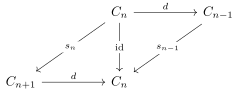Link to Diagram

Take \begin{align*} C = \qty{ 0 \to {\mathbb{Z}}/2{\mathbb{Z}}\xrightarrow{d} {\mathbb{Z}}/4{\mathbb{Z}}\to {\mathbb{Z}}/2{\mathbb{Z}}\to 0 } .\end{align*} Then $$\operatorname{im}d = \left\{{0, 2}\right\} = \ker d$$, but this does not split since $${\mathbb{Z}}/2{\mathbb{Z}}^2 \not\cong {\mathbb{Z}}/4{\mathbb{Z}}$$: one has an element of order 4 in the underlying additive group. Equivalently, there is no complement to the image. What might be familiar from algebra is $$ds = \operatorname{id}$$, but the more general notion is $$dsd = d$$.

The following complex is not split exact for the same reason: \begin{align*} \cdots \xrightarrow{\cdot 2} {\mathbb{Z}}/4{\mathbb{Z}}\xrightarrow{\cdot 2} {\mathbb{Z}}/4{\mathbb{Z}}\to \cdots .\end{align*}

Given $$f,g: C\to D$$, when do we get equality $$f_* = g_*: H_*(C) \to H_*(D)$$?

A chain map $$f:C\to D$$ is nullhomotopic if and only if there exist maps $$s_n: C_n\to D_{n+1}$$ such that $$f = ds + sd$$: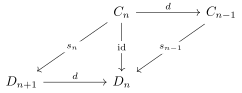Link to Diagram

The map $$s$$ is called a chain contraction. Two maps are chain homotopic (or initially: $$f$$ is chain homotopic to $$g$$, since we don’t yet know if this relation is symmetric) if and only if $$f-g$$ is nullhomotopic, i.e. $$f-g = ds + sd$$. The map $$s$$ is called a chain homotopy from $$f$$ to $$g$$. A map $$f$$ is a chain homotopy equivalence if both $$fg$$ and $$gf$$ are chain homotopic to the identities on $$C$$ and $$D$$ respectively.

If map $$f:C\to D$$ is nullhomotopic then $$f_*: H_*(C) \to H_*(D)$$ is the zero map. Thus if $$f,g$$ are chain homotopic, then they induce equal maps.

An element in the quotient $$H_n(C)$$ is represented by an $$n{\hbox{-}}$$cycle $$x\in Z_n(C)$$. By a previous exercise, $$f(x)$$ is a well-defined element of $$H_n(D)$$, and using that $$d(x) = 0$$ we have \begin{align*} f(x) = (ds + sd)(x) = d(s(x)) ,\end{align*} and so $$f[x] = [f(x)] = $$.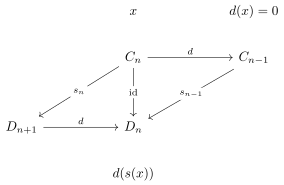Link to Diagram

Now applying the first part to $$f-g$$ to get the second part.

See Weibel for topological motivations.

## 6.2 1.5 Mapping Cones

Note that we’ll skip mapping cylinders, since they don’t come up until the section on triangulated categories. The goal is to see how any two maps between homologies can be fit into a LES. This helps reduce questions about quasi-isomorphisms to questions about split exact complexes.

Suppose we have a chain map $$f:B\to C$$, then there is a chain complex $$\operatorname{cone}(f)$$, the mapping cone of $$f$$, defined by \begin{align*} \operatorname{cone}(f)_n = B_{n-1} \oplus C_n .\end{align*}

The maps are given by the following:Link to Diagram

We can write this down: $$d(b, c) = (-d(b), -f(b) + d(c))$$, or as a matrix \begin{align*} \begin{bmatrix} -d^B & 0 \\ -f & d^C \end{bmatrix} .\end{align*}

Check that the differential on $$\operatorname{cone}(f)$$ squares to zero.

When $$f = \operatorname{id}:C\to C$$, we write $$\operatorname{cone}(C)$$ instead of $$\operatorname{cone}(\operatorname{id})$$. Show that $$\operatorname{cone}(C)$$ is split exact, with splitting map $$s(b, c) = (-c, 0)$$ for $$b\in C_{n-1}, c\in C_n$$.

Suppose $$f:B\to C$$ is a chain map, then the induced maps $$f_*: H(B) \to H(C)$$ fit into a LES. There is a SES of chain complexes:Link to Diagram

Check that these are chain maps, i.e. they commute with the respective differentials $$d$$.

The corresponding LES is given by the following:Link to Diagram

The map $${{\partial}}= f_*$$

Letting $$b\in B_n$$ is an $$n{\hbox{-}}$$cycle.

1. Lift $$b$$ to anything via $$\delta$$, say $$(-b, 0)$$.
2. Apply the differential $$d$$ to get $$(db, fb) = (0, fb)$$ since $$b$$ was a cycle.
3. Pull back to $$C_n$$ by the map $$C\to \operatorname{cone}(f)$$ to get $$fb$$.
4. Then the connecting morphism is given by $${{\partial}}[b] = [fb]$$. But by definition of $$f_*$$, we have $$[fb] = f_* [b]$$.

# 7 Friday, January 29

## 7.1 Mapping Cones

Given $$f:B\to C$$ we defined $$\operatorname{cone}(f)_n \mathrel{\vcenter{:}}= B_{n-1} \oplus C_n$$, which fits into a SES \begin{align*} 0 \to C \to \operatorname{cone}(f) \xrightarrow{\delta} B[-1] \to 0 \end{align*} and thus yields a LES in cohomology.Link to Diagram

$$f:B\to C$$ is a quasi-isomorphism if and only if $$\operatorname{cone}(f)$$ is exact.

In the LES, all of the maps $$f_*$$ are isomorphisms, which forces $$H_n(\operatorname{cone}(f)) = 0$$ for all $$n$$.

So we can convert statements about quasi-isomorphisms of complexes into exactness of a single complex.

We’ll skip the rest, e.g. mapping cylinders which aren’t used until the section on triangulated categories. We’ll also skip the section on $$\delta{\hbox{-}}$$functors, which is a slightly abstract language.

## 7.2 Ch. 2: Derived Functors

Setup: fix $$M\in {\mathsf{R}{\hbox{-}}\mathsf{Mod}}$$, where $$R$$ is a ring with unit. Note that by an upcoming exercise, $$\mathop{\mathrm{Hom}}_{R}(M, {-}): {\mathsf{Mod}{\hbox{-}}\mathsf{R}}\to {\mathsf{Ab}}$$ is a left-exact functor, but not in general right-exact: given a SES \begin{align*} 0\to A \xrightarrow{f} B \xrightarrow{g} C\to 0 && \in \mathsf{Ch}({\mathsf{Mod}{\hbox{-}}\mathsf{R}}) ,\end{align*} there is an exact sequence: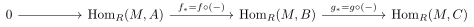Link to Diagram

However, this is not generally surjective: not every $$M\to C$$ is given by composition with a morphism $$M\to B$$ (lifting). To create a LES here, one could use the cokernel construction, but we’d like to do this functorially by defining a sequence functors $$F^n$$ that extend this on on the right to form a LES: